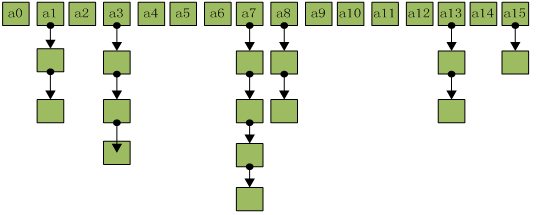``````HashMap<String, Float> map = new HashMap<String, Float>();
map.put("语文", 86.5f);
map.put("数学", 93.0f);
map.put("英语", 90.0f);
``````

#### HashMap存储结构图

HashMap采取数组加链表的存储方式来实现。亦即数组（散列桶）中的每一个元素都是链表，如下图：`PS:以下源码基于JDK1.7`

#### HashMap类的put(K key, V value)方法的源代码如下。

``````public V put(K key, V value) {
if (table == EMPTY_TABLE) {
inflateTable(threshold);
}
//如果key为null，调用putForNullKey方法进行处理
if (key == null)
return putForNullKey(value);
//根据key计算Hash值
int hash = hash(key);
//搜索指定hash值在对应table中的索引
int i = indexFor(hash, table.length);
//如果i索引处的Entry不为null，通过循环不断遍历e元素的下一个元素
for (Entry<K,V> e = table[i]; e != null; e = e.next) {
Object k;
//找到指定key与需要放入的key相等(hash值相同，通过equals比较放回true)
if (e.hash == hash && ((k = e.key) == key || key.equals(k))) {
V oldValue = e.value;
e.value = value;
e.recordAccess(this);
return oldValue;
}
}
//如果i索引处的Entry为null，表明此处还没有Entry
modCount++;
//将key、value添加到i索引处
return null;
}
``````

``````void addEntry(int hash, K key, V value, int bucketIndex) {
// 如果Map中的key-value对的数量超过了极限 并且bucketIndex索引处的table不为null
if ((size >= threshold) && (null != table[bucketIndex])) {
//把table对象的长度扩容2倍
resize(2 * table.length);
hash = (null != key) ? hash(key) : 0;
bucketIndex = indexFor(hash, table.length);
}

createEntry(hash, key, value, bucketIndex);
}
``````

• `size`：该变量保存了该HashMap中所包含的key-value对的数量
• `threshold`: 该变量包含了HashMap能容纳的key-value对的极限，它的值等于HashMap的容量乘以负载因子(load factor)

• `HashMap(): 构建一个初始容量为16，负载因子为0.75的HashMap`
• `HashMap(int initialCapacity)：构建一个初始容量为initialCapacity，负载因子为0.75的HashMap`
• `HashMap(int initialCapacity, float loadFactor)：以指定初始容量、指定的负载因子创建一个HashMap`

``````public HashMap(int initialCapacity, float loadFactor) {
if (initialCapacity < 0)
throw new IllegalArgumentException("Illegal initial capacity: " +
initialCapacity);
if (initialCapacity > MAXIMUM_CAPACITY)
initialCapacity = MAXIMUM_CAPACITY;
throw new IllegalArgumentException("Illegal load factor: " +

threshold = initialCapacity;  //①   计算阈值，这句是重点
init();
}
``````

``````public HashMap(int initialCapacity, float loadFactor) {
if (initialCapacity < 0)
throw new IllegalArgumentException("Illegal initial capacity: " +
initialCapacity);
if (initialCapacity > MAXIMUM_CAPACITY)
initialCapacity = MAXIMUM_CAPACITY;
throw new IllegalArgumentException("Illegal load factor: " +

// Find a power of 2 >= initialCapacity
int capacity = 1;
while (capacity < initialCapacity)
capacity <<= 1;

threshold = (int)(capacity * loadFactor); // 计算阈值 JDK1.6
table = new Entry[capacity];
init();
}
``````

``````threshold = (int)(capacity * loadFactor);
``````

``````threshold = (int)(16 * 0.75) = 12
``````

#### HashMap类的get(K key)方法代码如下。

``````public V get(Object key) {
//如果key时null，调用getForNullKey取出对应的value
if (key == null)
return getForNullKey();
//调用getEntry()方法
Entry<K,V> entry = getEntry(key);

return null == entry ? null : entry.getValue();
}

final Entry<K,V> getEntry(Object key) {
if (size == 0) {
return null;
}
//根据key的hashCode值计算它的hash码
int hash = (key == null) ? 0 : hash(key);
//直接取出table数组中指定索引处的值
for (Entry<K,V> e = table[indexFor(hash, table.length)];
e != null;
//搜索该Entry链的下一个Entry
e = e.next) {
Object k;
//如果该Entry的key与被搜索key相同,返回对应的value
if (e.hash == hash &&
((k = e.key) == key || (key != null && key.equals(k))))
return e;
}
return null;
}
``````

HashMap在底层将key-value当成一个整体进行处理，这个整体就是一个Entry对象。HashMap底层采用一个Entry[]数组来保存所有的key-value对，当需要存储一个Entry对象时，会根据Hash算法来决定其存储位置；当需要取出一个Entry时，也会根据Hash算法找到其存储位置，直接取出该Entry。由此可见，HashMap之所以能快速存、取它所包含的Entry，完全类似于现实生活中的：不同的东西要放在不同的位置，需要时才能快速找到它。